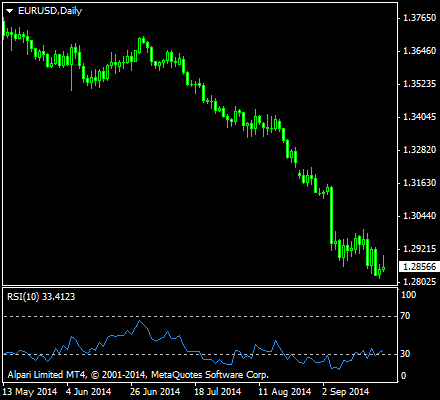# Relative Strength Index

The Relative Strength Index or RSI is a type of technical analysis indicator used in trading. It is a momentum indicator, meaning that it shows the strength of the price of a certain asset, thus the “strength” in its name. Momentum is the rate at which a price rises or falls. RSI compares the values of recent gains to the values recent losses in an attempt to determine overbought and oversold conditions of an asset.

The RSI was developed by J. Welles Wilder, an American mechanical engineer. He is also credited with other technical analysis indicators including the Average Directional Index, and the Average True Range just to name a few. He intended to chart the current and historical strength or weakness of a stock or market based on the closing prices of a recent trading period. His 1978 book entitled New Concepts in Technical Trading Systems has made RSI a popular oscillator index.

The RSI allows for traders to see the relative strength or momentum of an asset price.The RSI calculates the momentum as the ratio of higher closes to lower closes. Stocks that have had stronger positive changes have a higher RSI than stocks which have had more negative changes. This makes the RSI to be a momentum oscillator. It measures the speed and direction of price movements.

RSI is often used in mid-term timeframes, normally using 14-day periods. The RSI is measured in a scale from 0 to 100, with a high level at 70 and a low level at 30. Traders use shorter or longer time frames for alternately shorter or longer outlooks. More extreme high and low levels such as 80 and 20, or 90 and 10 respectively, occur less frequently but indicate stronger momentum. These levels are therefore not often used by most traders.

## Formula for RSI

RSI is computed by taking the ratio of the up and down exponential moving averages for a certain period of time and apply it to an index formula. The formula is:

RSI = 100 — 100 / (1 + RS)

where RS is the ratio of the EMAs.

Taking the upward changes can be computed by the difference of the closing prices.

U = current close — previous close

and the same is true for downward changes or computation for lows:

D = current close — previous close

RS can now be computed as:

RS = EMA(U, n) / EMA(D, n)

where n is the number of periods chosen for the RSI.

Analysis of the Formula for RSI

Let us look again at the index formula for the RSI.

RSI = 100 — 100 / (1 + RS)

This is a strength index, which means that the closer RSI to 100, the stronger the price an asset is. Conversely, the closer it is to 0, the weaker it is. What could be the conditions for an RSI to have a high value? RSI can have a high value if the second term in its equation is smaller. That means, a value that is close to 0 will be deducted from the first term which is 100, and should result to an RSI close to 100, or a very high value.

If the second term of the formula must be very small to yield a high RSI, the denominator which includes RS should also be close to 100 for the whole term to yield a quotient of 0 — 1. If RS is close to 100, then 100/~100 = 0 to approximately 1. Computing for the whole value, that would be 100 — ~0 to 1 would yield a number very close to 100, hence interpreted as a high relative strength index.

Conversely, if RS is very low, say 0, then 1 + 0 in the denominator would yield 1, and the quotient of 100/1 would still be 100. Subtract 100 from the second term which is also 100 yields to an RSI of 0. It is then safe to say that RS and RSI are directly related, wherein the magnitude of these two values are very close or the same. Let’s keep this in mind as we deal next with this relationship in regards to the computation of moving averages for highs and lows.

If RSI is high, say 100, then we mentioned that RS must also be relatively high. A relatively high RS can only be derived from a very high EMA for upward movement in relation to a very low EMA for the downward movement. Conversely, for an RS to have a very low value, it is enough for the EMA of the downward movement to overcome the moving average of the upward movement for a certain time period. In the same manner, RSI is low here.## Overbought and Oversold

Signals can also be generated from RSI by looking for divergences, failure swings and centerline crossovers. RSI can also be used to identify the general trend. As traders can see from different stock charts, the RSI indicator that ranges from 0 to 100 is deemed to be overbought once the RSI approaches the 70 level. This means that it may be getting overvalued and is a good candidate for a pullback. Likewise, if the RSI approaches 30, it can be an indicator that the asset may be getting oversold and therefore likely to become undervalued.

There are many conditions for an asset to become overbought or oversold. Wilder proposed that when price moves up very rapidly, at some point, it will be considered overbought. Likewise, when price falls very rapidly, at some point, it will be considered oversold. In either case, these situations often react to imminent reversals. Traders use this as a signal to trade in the different direction, given all things equal.

The level of the RSI is a measure of the stock’s recent trading strength. The slope of the RSI is directly proportional to the velocity of a change in the trend. The displacement created by the RSI is proportional to the magnitude of the move. The tops and bottoms indicated by the 70 and 30 levels also define a territory of neutral level, one where the 50 level becomes a sign for traders that the asset is not trending.

Stay informed with more articles presented by us. In the meantime, why don’t you check out our list of best binary brokers, so that you can start trading? We are your partner in binary options success.